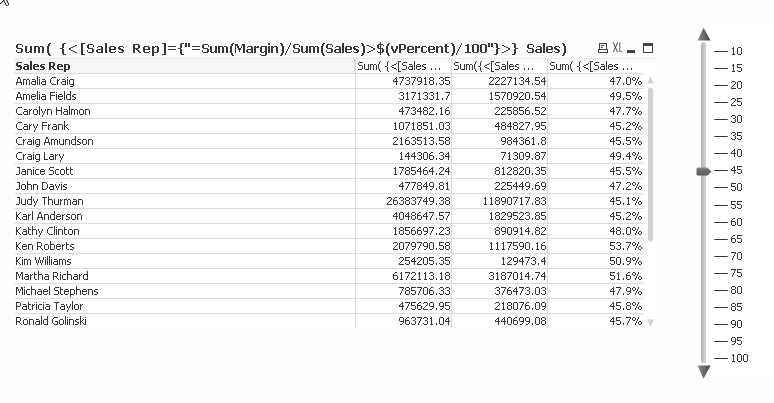# QlikView App Development

Discussion Board for collaboration related to QlikView App Development.

HighlightedPartner

## Hide rows in pivot table.

Hi All,

I have a pivot table with an alternate state.The user can select 2 different periods to compare.

I'va also created a slider.

Is there a way that I can only show the rows with a percentage higher or equal to the selected % in the slider?Regards,

Kris

Tags (2)
1 Solution

Accepted SolutionsMVP

## Re: Hide rows in pivot table.

It would have to not include the outer curly braces:

vPctAbove = '<[Sales Rep]={"=Sum(Margin)/Sum(Sales)>\$(vPercent)/100"}>'

Then you could do:

Sum( {State1\$(vPctAbove)} Quantity )

or maybe even a more reusable way:

vPctAbove = '[Sales Rep]={"=Sum(Margin)/Sum(Sales)>\$(vPercent)/100"}'

and

Sum( { State1 <\$(vPctAbove)> } Quantity )

5 RepliesMVP

## Re: Hide rows in pivot table.

Could be possible from 2 different selection with 2 different objects. Can you post example

Before develop something, think If placed (The Right information | To the right people | At the Right time | In the Right place | With the Right context)MVP

## Re: Hide rows in pivot table.

Yes by using a set expression in all the expressions:This the set expression which is similar for all expressions could be stored in a variable too so it is simpler to maintain and use in multiple expressions:

vPctAbove = '{<[Sales Rep]={"=Sum(Margin)/Sum(Sales)>\$(vPercent)/100"}>}'

Then the expression for Sum(Sales) should be Sum( \$(vPctAbove) Sales)

for Sum(Margin)/Sum(Sales) it wold be Sum( \$(PctAbove) Margin) / Sum(Sales)

The hiding relies on the fact that all rows which contains zeros in all the expressions will be excluded.

I did the test and example in the "Data Visualization" example QlikView app that comes with QlikView to illustrate and prove my point since you didn't provide any test data.Partner

## Re: Hide rows in pivot table.

Thanks Petter,

It works.

But how should I use the variable vPctAbove in my expression with the alternate state?

sum( {State1}  Quantity)MVP

## Re: Hide rows in pivot table.

It would have to not include the outer curly braces:

vPctAbove = '<[Sales Rep]={"=Sum(Margin)/Sum(Sales)>\$(vPercent)/100"}>'

Then you could do:

Sum( {State1\$(vPctAbove)} Quantity )

or maybe even a more reusable way:

vPctAbove = '[Sales Rep]={"=Sum(Margin)/Sum(Sales)>\$(vPercent)/100"}'

and

Sum( { State1 <\$(vPctAbove)> } Quantity )Partner

## Re: Hide rows in pivot table.

Thanks Petter!!

It is working fine for me.